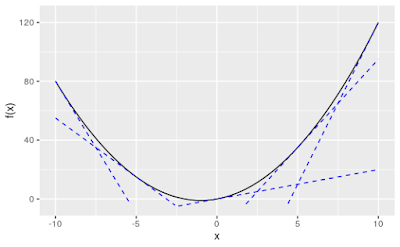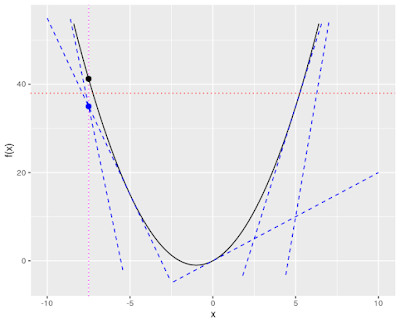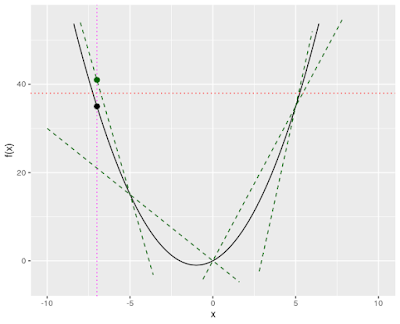## Friday, March 27, 2020

### A Minimum Variance Partition Problem

Someone posed a question on Mathematics Stack Exchange that I answered there. This post is to explain the model I used (and generalize a bit).

In general terms, you start with a collection $\Omega=[\omega_1, \dots, \omega_n]$ of positive integers. I choose to think of the integers as weights. The mission is to partition $\Omega$ into subcollections $S_1, \dots, S_m$ such that (a) you use as few subcollections as possible (i.e., minimize $m$), (b) the variance of their weight totals is minimal, and (c) no subcollection in the partition has total weight greater than some specified constant $L$. (If, for example, $S_1 = [\omega_4, \omega_9, \omega_{10}]$ then the weight total for $S_1$ is $\omega_4 + \omega_9 + \omega_{10}$.)  Why am I saying "collections" rather than sets? This would be a set partitioning problem were it not for the fact that $\Omega$ may contain duplicate entries and so is technically a multiset.

The term "variance" is slightly ambiguous (population variance or sample variance), but happily that will not matter here. Let $$s_j =\sum_{i : \omega_i \in S_j} \omega_i$$be the weight of subcollection $S_j$. Since we are talking about a partition here (every item in exactly one subcollection), $$\sum_{j=1}^m s_j = \sum_{i=1}^n \omega_i = n \bar{\omega}$$where $\bar{\omega}$ is the (constant) mean of all the item weights, and so the mean of the subcollection weights is $$\bar{s} = \frac{n\bar{\omega}}{m},$$which for a given number $m$ of subcollections is constant. The variance of the subcollection weights is $$\frac{1}{m}\sum_{j=1}^m (s_j - \bar{s})^2=\frac{1}{m}\sum_{j=1}^m s_j^2-\bar{s}^2.$$To minimize that, we can just minimize $\sum_j s_j^2$ in the optimization model, and then do the arithmetic to get the variance afterward.

As originally articulated, the problem has two objectives: minimize the variance of the subcollection weights and minimize the number of subcollections used. Normally, a multiobjective problem involves setting priorities or assigning weights to objectives or something along those lines. For the original question, where there are only $n=12$ elements in $\Omega$ (and thus at most $m=12$ sets in the partition), a plausible approach is to find a minimum variance solution for each possible value of $m$ and present the user with the efficient frontier of the solution space, letting the user determine the trade-off between partition size and variance that most appeals to them. For the particular example in the SE question, I was a bit surprised to find that the variance increased monotonically with the number of sets in the partition, as seen in the following table.

 # of Subcollections Minimum Variance 1 infeasible 2 infeasible 3 infeasible 4 infeasible 5 672 6 1048.22 7 1717.39 8 3824.75 9 7473.73 10 9025.80 11 9404.81 12 11667.06

So the five subcollection partition is minimal both in number of subcollections and weight variance. I would be surprised if that generalized.

The QP formulation uses two sets of variables. For $i=1,\dots,n$ and $j=1,\dots,m$, $x_{ij}\in \lbrace 0, 1\rbrace$ determines whether $\omega_i$ belongs to $S_j$ ($x_{ij}=1$) or not ($x_{ij}=0$). For $j=1,\dots,m$, $s_j \ge 0$ is the total weight of subcollection $j$. The objective function is simply $$\min_{x,s} \sum_{j=1}^m s_j^2$$which happily is convex. The first constraint enforces the requirement that the subcollections form a partition (every element being used exactly once): $$\sum_{j=1}^m x_{ij} = 1 \quad\forall i\in \lbrace 1,\dots,n\rbrace.$$For a partition to be valid, we do not want any empty sets, which leads to the following constraint:$$\sum_{i=1}^n x_{ij} \ge 1\quad\forall j\in\lbrace 1,\dots,m\rbrace.$$ The next constraint defines the weight variables: $$s_j = \sum_{i=1}^n \omega_i x_{ij}\quad\forall j\in\lbrace 1,\dots,m\rbrace.$$What about the limit $L$ on the weight of any subcollection? We can enforce that by limiting the domain of the $s$ variables to $s_j\in [0,L]$ for all $j$.

Finally, there is symmetry in the model. One source is duplicate items in $\Omega$ ($\omega_i = \omega_{i'}$ for some $i\neq i'$). That happens in the data of the SE question, but it's probably not worth dealing with in an example that small. Another source of symmetry is that, given any partition, you get another partition with identical variance by permuting the indices of the subcollections. That one is easily fixed by requiring the subcollections to be indexed in ascending (or at least nondecreasing) weight order:$$s_j \le s_{j+1}\quad\forall j\in\lbrace 1,\dots,m-1\rbrace.$$Is it worth bothering with? With the anti-symmetry constraint included, total run time for all 12 models was between one and two seconds on my PC. Without the anti-symmetry constraint, the same solutions for the same 12 models were found in a bit over 100 minutes.

If you would like to see my Java code (which requires CPLEX), you can find it at https://gitlab.msu.edu/orobworld/mvpartition.

## Tuesday, March 24, 2020

### Approximating Nonlinear Functions: Tangents v. Secants

In a (much) earlier post , I discussed using SOS2 constraints to approximate a nonlinear function in a mixed-integer linear program (MILP). In this post, and probably the next one or two, I'm going to expand on that theme a bit. (By "a bit" I mean "endlessly".)

### Summary

To save you some reading, in case you end up not interested, I'll summarize the take-aways here.
1. We can approximate nonlinear functions in a MILP model using piecewise-linear functions based on either tangents or secants. Piecewise-linear functions implicitly add either binary variables or SOS2 constraints (or maybe both).
2. For convex functions only appearing in $\le$ constraints or concave functions only appearing in $\ge$ constraints, we can say that using tangents may produce superoptimal solutions (look optimal but are actually infeasible) but will not produce suboptimal solutions, while using secants may produce suboptimal solutions but will not produce infeasible solutions. In any other case, super- and sub-optimality are both risks.
3. For the same two combinations in the previous paragraph, we have a third option: using a set of linear inequalities based on tangents, rather than a piecewise-linear function. Besides being somewhat easier to set up, this has a potential advantage: using a callback, we can refine the approximation on the fly (by adding additional constraints based on new tangents as they are needed).

### Gory details

Suppose that we are working on a MILP, and we need to incorporate a nonlinear function of some of the variables. To keep things simple, I'll illustrate with functions $f:\mathbb{R}\rightarrow\mathbb{R}$ of a single variable, but the concepts presented will extend (not necessarily easily) to functions of multiple variables. In what follows, I will refer to the set of points $(x,f(x))$ as the surface of $f$. A tangent to the surface at $x$ is a line (more generally, hyperplane) that touches the surface at $x$ and has the same slope as the surface at that one point. A secant to the surface is a line (more generally, hyperplane) that intersects the surface at multiple points. For a function of one dimension, a chord is a segment of a secant between two consecutive intersection points. ("Consecutive" means that there are no other intersections of secant and surface between the endpoints of the chord.) I'm not sure if the term "chord" is used in higher dimensions, although the concept extends to higher dimensions.

Figure 1 shows a convex function together with a few tangents (in blue).Figure 1: Tangents to convex function /* Layout-provided Styles */ div.standard { margin-bottom: 2ex; }
Figure 2 shows the same function with a few secants (in green). One of the secants intersects the surface at $(-5,15)$ and $(0,0)$. The dashed line segment between those points is a chord.Figure 2: Secants to convex function
Figures 3 and 4 do the same for a concave function.Figure 3: Tangents to concave functionFigure 4: Secants to concave function

A useful property of convex and concave functions is that tangents of convex functions are always less than or equal to the functions and tangents of concave functions are always greater than or equal to the functions. Similar claims cannot be made for secants. A chord of a convex function will always be greater than or equal to the function, but other points on its secant can be less than the function. For instance, in Figure 2 the chord from $x=-5$ to $x=0$ lies above the function surface, but the secant to which it belongs falls below the function surface for $x<-5$ or $x>0$. It is worth noting that tangents to nonconvex functions do not stay on one side of the surface. Figure 5 shows a nonconvex function along with one tangent (in blue) and one secant (in green). Both the tangent and the secant are above the function surface some places and below it other places.Figure 5: Nonconvex function

In what follows, when I talk about convexity of the feasible region, I will be referring to the feasible region of the continuous relaxation (all integrality restrictions relaxed). Convexity of that region is critical to algorithms using LP bounds. There are two cases of initial interest, because they preserve that convexity. If the function $f()$ is convex and appears only in constraints of the form $f(x)+\dots\le b$ (where $\dots$ is empty or contains only convex terms), or is concave and appears only in constraints of the form $f(x)+\dots\ge b$ (where $\dots$ is empty or contains only concave terms), and if the other constraints do nothing to violate convexity, then the feasible region remains convex when $f()$ is introduced. Under any other scenario, you lose convexity of the feasible region when you introduce $f()$. So for now we will only consider convex $f()$ in $\le$ constraints or concave $f()$ in $\ge$ constraints. If $f()$ is convex or concave but appears in an inequality constraint with the wrong direction, or appears in an equation constraint, or if $f()$ is neither convex nor concave, we will deal with it later (or never, whichever comes second).

For simplicity, suppose that $f()$ is convex. We introduce a new variable $z$ that is a surrogate for $f(x)$, changing each constraint of the form $f(x)+\dots\le b$ to $z+\dots\le b$. Now we have to tie $z$ to $f(x)$, but without using the explicit constraint $z=f(x)$ (due to that whole nonlinearity thing). We can approximate the relationship either with tangents or with secants. With secants, we pick an arbitrary collection of values of $x$, calculate the corresponding values of $f(x)$, define a piecewise-linear function $\hat{f}(x)$ based on those values, and set $z=\hat{f}(x)$. In Figure 2, $\hat{f}(x)$ consists of the four chords shown (with endpoints at $x\in\left\{ -10,-5,0,5,10\right\}$).

With tangents, we have a choice. Both options involve computing tangents at an arbitrary collection of values of $x$. In one version, we find where those tangents intersect and use the intersection points to define a piecewise-linear function. In Figure 1, the five tangents shown intersect at $\left\{ (-7.5,35),(-2.5,-5),(2.5,5),(7.5,65)\right\}$. We can add endpoints for where the first and last tangents exit the interval of interest (say $(-10,80)$ and $(10,120)$ for Figure 1). In the other version, we add the constraints $z\ge\ell_{j}(x)$ for $j=1,\dots,t$, where $t$ is the number of tangents computed and $\ell_{1}(x),\dots,\ell_{t}(x)$ are the linear functions corresponding to the tangents. In Figure 1, $t=5$ and for any $x$ we are constraining $z$ to be greater than or equal to the ordinates of all five dotted lines at that $x$. If $f()$ is concave and appears in the constraint $f(x)\ge b$, the only change is that the added constraints are $z\le\ell_{j}(x)$.

At this point, there are several things we can note.
1. Calculating chords only requires the evaluation of $f(x)$ for various values of $x$ (plus some simple algebra). Calculating tangents requires computing the first derivative of $f(x)$ when $f()$ is smooth. (More generally, it involves calculating the subgradient of $f()$ at $x$.) It then requires calculating where the tangents intersect, if we are going to make a piecewise-linear function from the tangents. So if $f()$ is painful to work with, or if sorting out a lot of intersections does not appeal to you, you might prefer chords.
2. Chords involve embedding a piecewise-linear function in a model. As discussed in , this can be done using type two special ordered sets (SOS2), which turns an LP into a MIP and turns a MIP into a somewhat more complicated MIP. In another post , I discussed a handy feature in the CPLEX APIs that allows for easy entry of a piecewise-linear function. (In the Java API, the method is IloCplexModeler.piecewiseLinear(), which in turn implements a method from the IloMPModeler  interface.) The API method still introduces either binary variables or SOS2 constraints "under the hood", but at least the user is spared the tedious business of computing them. With tangents, the first option described does the same thing, but the second option just involves adding a bunch of linear constraints. (In CPLEX, if you calculate a lot of tangents, you might consider adding the constraints as "lazy constraints".)
3. The way the approximations affect the solution differs between tangents and secants.
1. In Figures 1 and 3, it is easy to see that if we replace $f(x)$ with a finite set of tangents, every feasible point remains feasible, but some infeasible points appear feasible. For instance, take the constraint $f(x)\le38$ (which becomes $z\le38$) with the function in Figure 1, redrawn below in Figure 6. The horizontal dotted line (in red) shows the limit (38) imposed by the constraint. The vertical dotted line (in cyan) shows what happens when $x=-7.5$. The black point, $(-7.5,f(-7.5))$ is above the red dotted line, indicating that $x=-7.5$ is infeasible. The blue point, $(-7.5,35)$, is however feasible in the relaxed problem, since it is on or above all the selected tangents and below the constraint limit. So the solver might accept a solution containing $x=-7.5$.
2. In Figures 2 and 4, it is likewise easy to see that if we replace $f(x)$ with a piecewise-linear function consisting of chords, solutions that are feasible in the relaxed problem will also be feasible in the original problem, but "optimal" solutions to the relaxed problem may actually be suboptimal. The secant approximation to our convex function in Figure 2 is redrawn below in Figure 7. Staying with the constraint $z\le38$, consider what happens at $x=-7$ (the dotted vertical line). The actual function value $f(-7)=35$ is below the constraint limit, so $x=-7$ satisfies the constraint. (This is the black point on the graph.) Barring exclusion due to other constraints, it would be feasible and might be part of an optimal solution. The green point $(-7,41)$, on the piecewise-linear function defined by the secants, is not feasible, however, and so the solver will reject any solution containing $x=-7$, including possibly the true optimum.Figure 6: Infeasible pointFigure 7: Feasible point excluded

Since tangents may produce infeasible "solutions" and secants may produce suboptimal solutions, we may need to solve the problem, get a sense of where the optimal solution might be located, refine the approximation near there and repeat. Note that if we use tangents for convex or concave functions (which produces an "outer approximation" to the original problem) and the final solution is really feasible (using the actual $f()$), there is no need to refine further. Unfortunately, with secants, I see no obvious way to know that our approximation is "good enough". With functions that are neither convex nor concave, or with convex/concave functions in equations or inequalities going the "wrong" direction, tangents no longer provide an outer approximation (Figure 5), and we may need to refine and repeat.

This brings me to my last point, which pertains to the cases where tangents do provide an outer approximation and where we add all the tangents as constraints rather than turning them into a piecewise-linear function. With solvers, like CPLEX, that allow users to post new constraints during the branch-and-bound process, we can refine on the fly. Referring back to Figure 6, suppose that I am solving the problem using CPLEX, with a lazy constraint callback attached, and CPLEX identifies a solution containing $x=-7.5$. Inside the callback, I can compute $f(-7.5)$, observe that it is greater than the constraint cutoff of $38$ (and also greater than $z=35$), calculate a new tangent at the black point, and add it as a lazy constraint. This will make the proposed new incumbent infeasible. Figure 8 shows this, with the orange dashed line being the tangent at $x=-7.5$. After adding it to our set of tangents (and adding the constraint $z\ge\ell(x)$, where $\ell(x)$ is the linear function given by the new tangent), the blue point no longer gives a possible value for $z$. At $x=-7.5$, the maximum value of any tangent will be the value of $\ell(-7.5)$, and that will equal $f(-7.5)$ since we calculated the tangent there. (It's hard to see in the figure, but trust me, the orange line goes through the black point.) So the solver will update $z$ to the correct value and, in this case, reject the solution as violating the constraint $z\le38$.Figure 8: Adding a tangent

### References

 "Linearize That!"
 "Piecewise Linear Functions in CPLEX"